## Example Questions

← Previous 1

### Example Question #1 : How To Find The Midpoint Of A Line Segment

What is the coordinate of the point that is halfway between (-2, -4) and (6, 4)?

(2,2)

(3,1)

(0,2)

(2,0)

(2,0)

Explanation:

The midpoint formula is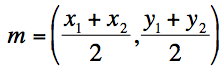### Example Question #1 : How To Find The Midpoint Of A Line Segment

What is the midpoint of MN between the points M(2, 6) and N (8, 4)?

(2, 1)

(5, 5)

(5, 2)

(3, 1)

(3, 5)

(5, 5)

Explanation:

The midpoint formula is equal to. Add the x-values together and divide them by 2, and do the same for the y-values.

x: (2 + 8) / 2 = 10 / 2 = 5

y: (6 + 4) / 2 = 10 / 2 = 5

The midpoint of MN is (5,5).

### Example Question #3 : How To Find The Midpoint Of A Line Segment

In the standard coordinate plane, what is the midpoint of a line segment that goes from the point (3, 5) to the point (7, 9)?

(6,6)

(–2, –2)

(7, 5)

(10,14)

(5, 7)

(5, 7)

Explanation:

The midpoint formula is. An easy way to remember this is that finding the midpoint simply requires that you find the averageof the two x-coordinates and the average of the two y-coordinates. In this case, the two x-coordinates are 3 and 7, and the two y-coordinates are 5 and 9. If we substitute these values into the midpoint formula, we get (3 + 7/2), (5 + 9)/2, which equals (5, 7). If you got (–2, –2), you may have subtracted your x and y-coordinates instead of adding. If you got (10,14), you may have forgotten to divide your x and y-coordinates by 2. If you got (6,6), you may have found the average of x1 and y2 and x2 and y1 instead of keeping the x-coordinates together and the y-coordinates together. If you got (7, 5), you may have switched the x and y-coordinates.

### Example Question #4 : How To Find The Midpoint Of A Line Segment

Find the midpoint of a line segment with endpoints (–1, 4) and (3, 6).

(1, 5)

(5, 1)

(3, 2)

(4, 5)

(1, 5)

Explanation:

The formula for midpoint = (x1 + x2)/2, (y1 + y2)/2. Substituting in the two x coordinates and two y coordinates from the endpoints, we get (–1 + 3)/2.

(4 + 6)/2 or (1, 5) as the midpoint.

### Example Question #5 : How To Find The Midpoint Of A Line Segment

In the standard x, y coordinate plane what are the coordinates of the midpoint of a line whose endpoints are (–6, 4) and (4, –6)?

–1, –1

1, 1

1, –1

–1, –1/2

–1, 1

–1, –1

Explanation:

To solve this problem we use the midpoint formula.  We find the average of the x and y coordinates.  (–6 + 4)/2, (4 + –6)/2 = –1, –1

### Example Question #6 : How To Find The Midpoint Of A Line Segment

What is the midpoint of a real number line with points at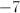and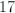?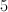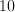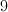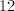Explanation:

Method A:

To find the midpoint, draw the number line that contains pointsand.Then calculate the distance between the two points.  In this case, the distance betweenandis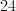.  By dividing the distance between the two points by 2, you establish the distance from one point to the midpoint.  Since the midpoint is 12 away from either end, the midpoint is 5.Method B:

To find the midpoint, use the midpoint formula: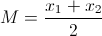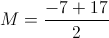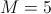### Example Question #1 : How To Find The Midpoint Of A Line Segment

Janice and Mark work in a city with neatly gridded streets. If Janice works at the intersection of 33rd Street and 7th Avenue, and Mark works at 15th Street and 5th Avenue, how many blocks will they each travel to lunch if they meet at the intersection exactly in between both offices?Explanation:

Translating the intersections into points on a graph, Janice works at (33,7) and Mark works at (15,5). The midpoint of these two points is found by taking the average of the x-coordinates and the average of the y-coordinates, giving ((33+15)/2 , (5+7)/2) or (24, 6). Traveling in one direction at a time, the number of blocks from either office to 24th street is 9, and the number of blocks to 6th is 1, for a total of 10 blocks.

### Example Question #8 : How To Find The Midpoint Of A Line Segment

On the real number line, what is the midpoint between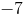and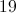?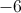Explanation:

On the number line,is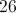units away from.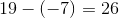We find the midpoint of this distance by dividing it by 2.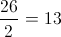To find the midpoint, we add this value to the smaller number or subtract it from the larger number.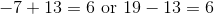The midpoint value will be.

### Example Question #9 : How To Find The Midpoint Of A Line Segment

What is the midpoint between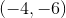and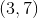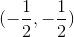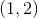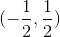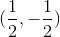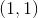Explanation:

Using the midpoint formula,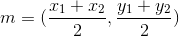We get: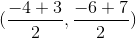Which becomes: which becomes### Example Question #10 : How To Find The Midpoint Of A Line Segment

What is the midpoint of a line segment with endpoints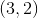and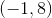?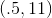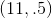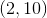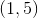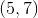Explanation:

The midpoint of a line can be found using the midpoint formula, given by: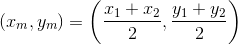Thus when we plug in our values we get the midpoint is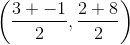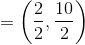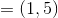← Previous 1